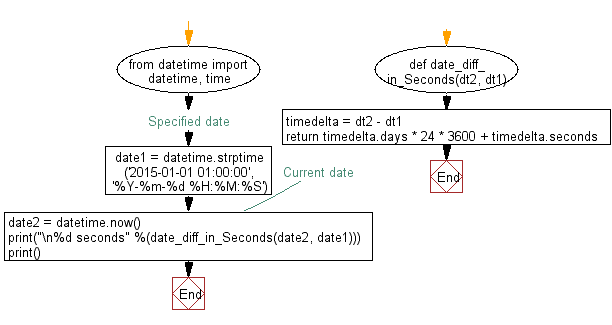﻿ Python: Calculate two date difference in seconds - w3resource# Python: Calculate two date difference in seconds

## Python Datetime: Exercise-36 with Solution

Write a Python program to calculate two date difference in seconds.

Sample Solution:

Python Code:

``````from datetime import datetime, time
def date_diff_in_Seconds(dt2, dt1):
timedelta = dt2 - dt1
return timedelta.days * 24 * 3600 + timedelta.seconds
#Specified date
date1 = datetime.strptime('2015-01-01 01:00:00', '%Y-%m-%d %H:%M:%S')
#Current date
date2 = datetime.now()
print("\n%d seconds" %(date_diff_in_Seconds(date2, date1)))
print()
```
```

Sample Output:

```74175795 seconds
```

Flowchart:Python Code Editor:

What is the difficulty level of this exercise?

Test your Python skills with w3resource's quiz

﻿

## Python: Tips of the Day

Free up Memory:

• Manual garbage collection can be performed on timely or event based mechanism.
```import gc
collected_objects = gc.collect()
```